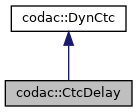codac
codac::CtcDelay Class Reference

$\mathcal{C}_{delay}$ that contracts the tubes $[x](\cdot)$ and $[y](\cdot)$ with respect to their delay $[\tau]$ according to the delay constraint $\mathbf{x}(t)=\mathbf{y}(t+\tau)$ More...

#include <codac_CtcDelay.h>

Inheritance diagram for codac::CtcDelay:[legend]
Collaboration diagram for codac::CtcDelay:[legend]

## Public Member Functions

CtcDelay ()
Creates a contractor object $\mathcal{C}_{delay}$.

void contract (Interval &a, Tube &x, Tube &y)
$\mathcal{C}_{delay}\big([a],[x](\cdot),[y](\cdot)\big)$: contracts the tubes $[x](\cdot)$, $[y](\cdot)$ and the delay $[a]$ with respect to the constraint $x(t)=y(t+a)$ More...

void contract (Interval &a, TubeVector &x, TubeVector &y)
$\mathcal{C}_{delay}\big([a],[\mathbf{x}](\cdot),[\mathbf{y}](\cdot)\big)$: contracts the tube vectors $[\mathbf{x}](\cdot)$, $[\mathbf{y}](\cdot)$ and the delay $[a]$ with respect to the constraint $\mathbf{x}(t)=\mathbf{y}(t+a)$ More...Public Member Functions inherited from codac::DynCtc
DynCtc (bool intertemporal=true)
Creates a contractor. More...

virtual ~DynCtc ()
DynCtc destructor.

void preserve_slicing (bool preserve=true)
Specifies whether the contractor can impact the tube's slicing or not. More...

void set_fast_mode (bool fast_mode=true)
Specifies an optional fast mode of contraction. More...

void restrict_tdomain (const Interval &tdomain)
Limits the temporal domain of contractions. More...

bool is_intertemporal () const
Tests if the related constraint is inter-temporal or not. More...

## Static Protected Attributes

static const std::string m_ctc_name
class name (mainly used for CN Exceptions)

static std::vector< std::string > m_str_expected_doms
allowed domains signatures (mainly used for CN Exceptions)Protected Attributes inherited from codac::DynCtc
bool m_preserve_slicing = true
if true, tube's slicing will not be affected by the contractor

bool m_fast_mode = false
some contractors may propose more pessimistic but faster execution modes

Interval m_restricted_tdomain
limits the contractions to the specified temporal domain

const bool m_intertemporal = true
defines if the related constraint is inter-temporal or not (true by default)

## Detailed Description

$\mathcal{C}_{delay}$ that contracts the tubes $[x](\cdot)$ and $[y](\cdot)$ with respect to their delay $[\tau]$ according to the delay constraint $\mathbf{x}(t)=\mathbf{y}(t+\tau)$

## ◆ contract() [1/2]

 void codac::CtcDelay::contract ( Interval & a, Tube & x, Tube & y )

$\mathcal{C}_{delay}\big([a],[x](\cdot),[y](\cdot)\big)$: contracts the tubes $[x](\cdot)$, $[y](\cdot)$ and the delay $[a]$ with respect to the constraint $x(t)=y(t+a)$

Parameters
 a the delay value $\tau$ to be contracted x the scalar tube $[x](\cdot)$ to be contracted y the scalar tube $[y](\cdot)$ to be contracted

## ◆ contract() [2/2]

 void codac::CtcDelay::contract ( Interval & a, TubeVector & x, TubeVector & y )

$\mathcal{C}_{delay}\big([a],[\mathbf{x}](\cdot),[\mathbf{y}](\cdot)\big)$: contracts the tube vectors $[\mathbf{x}](\cdot)$, $[\mathbf{y}](\cdot)$ and the delay $[a]$ with respect to the constraint $\mathbf{x}(t)=\mathbf{y}(t+a)$

Parameters
 a the delay value $\tau$ to be contracted x the n-dimensional tube $[\mathbf{x}](\cdot)$ to be contracted y the n-dimensional tube $[\mathbf{y}](\cdot)$ to be contracted

The documentation for this class was generated from the following file: